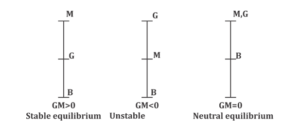Engineering Jobs   »   Civil Engineering quizs   »   DFCCIL QUIZ

# DFCCIL’21 CE: Daily Practice Quiz. 13-September-2021

DFCCIL (Dedicated Freight Corridor Corporation of India Limited) Exam Date 2021 on September and October 2021 so boost your preparation by attempt this Civil Engineering Hydraulics & Fluid Mechanics quiz for DFCCIL recruitment 2021.Quiz: Civil Engineering
Exam: DFCCIL-JE
Topic: Hydraulics & Fluid Mechanics

Each question carries 1 mark
Negative marking: 1/4 mark
Time: 8 Minutes

Q1. The rise or fall of head ‘h’ in a capillary tube of diameter ‘d’ and liquid surface tension σ and specific weight w is equal to
(a) 4σ\wd
(b) 4dσ/w
(c) 4wd\σ
(d) 4wσ\d

Q2. A liquid compressed in cylinder has a volume of 0.04 m³ at 50 N/cm² and a volume of 0.039 m³ at 150 N/cm². The bulk modulus of elasticity of liquid is:
(a) 400 N/cm²
(b) 4000 N/cm²
(c) 40000 N/cm²
(d) 40 N/cm²

Q3. The viscosity of a gas
(a) decreases with increase in temperature
(b) increases with increases in temperature
(c) is independent of temperature
(d) is independent of pressure for very high pressure intensities

Q4. One torr pressure is equivalent to:
(a) 1 atmosphere
(b) 1 mm of mercury
(c) 10 m of water
(d) 1 Pascal

Q5. The pressure in Pascals at a depth of 1 m below the free surface of a body of water will be equal to
(a) 1 Pa
(b) 98.1 Pa
(c) 981 Pa
(d) 9810 Pa

Q6. A body floats in stable equilibrium
(a) when its metacentric height is zero
(b) when the Meta Centre is above CG.
(c) when its CG is below its Centre of buoyancy
(d) Meta Centre has nothing to do with position of CG for determining stability

SOLUTION

S1. Ans.(a)
Sol. Capillary rise or fall
h = 4σcosθ/ρgd
But ω (Weight density) = ρg,
and cos θ for water and glass = 1
∵ ▭(h=4σ/ωd)

S2. Ans.(b)
Sol. Bulk modulus
K = – ∆P/(∆V/V)
K= -[(0.04×[150-50])/(0.039-0.04)]=4000 N\/cm^2

S3. Ans.(b)
Sol. As we know that
⇒ With increasing temp. viscosity of liquid decrease because as we increase the temperature which cause decreases the cohesive forces between modulus so that viscosity is decrease
⇒ with increasing temp. viscosity of gas increases because as we increase the temp. which cause increase the rate of molecules momentum transfer which is the main reason of viscous forces within the gases. So that viscosity in increase.

S4. Ans.(b)
Sol. 760 torr = 1 atmospheric pressure
760 mm = 1 atmospheric pressure
i.e. 1 mm of mercury = 760 torr
it is on the name of Torricelli

S5. Ans.(d)
Sol. Pressure (P) = ρ.gh
= 1000×9.81×1
= 9810 Pa.

S6. Ans.(b)
Sol.M = Metacenter
G= Center of gravity
B = center of buoyancy.
▭(GM=BM-BG)

Sharing is caring!

Thank You, Your details have been submitted we will get back to you.